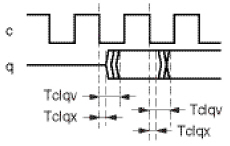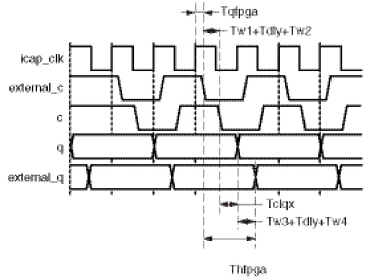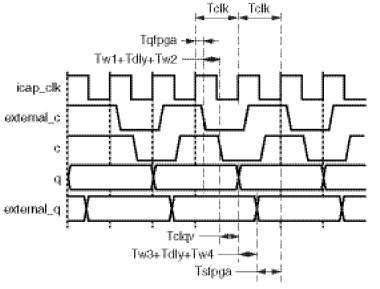# SPI Bus Receive Waveform and Timing Budget - 4.1 English

## Soft Error Mitigation Controller Product Guide (PG036)

Document ID
PG036
Release Date
2022-05-04
Version
4.1 English

The SPI flash device exhibits certain output switching characteristics of its output data with respect to its input clock. This analysis is for data capture at the system-level design example, when receiving data from the SPI flash device.

 X-Ref Target - Figure 3-10The following parameters, shown in This Figure , are defined as the output switching behavior of the SPI flash device:

T clqv = SPI flash maximum output valid with respect to clock

T clqx = SPI flash minimum output hold with respect to clock

The analysis assumes minimum propagation delays are zero. This analysis also assumes the following skews are negligible:

Skew on input clock distribution to FPGA output and input flip-flops.

Skew in PCB level translator channel delays. The level translator on clock and datapaths must be matched for this to be true.

Duty cycle distortion.

The following parameters are defined as implementation parameters of the EXT shim and PCB:

T clk = input clock cycle time ( icap_clk )

T qfpga = FPGA output delay with respect to icap_clk

T sfpga = FPGA input setup requirement with respect to icap_clk

T hfpga = FPGA input hold requirement with respect to icap_clk

T w1 = FPGA to level translator PCB trace delay

T w2 = Level translator to SPI flash PCB trace delay

T w3 = SPI flash to level translator PCB trace delay

T w4 = Level translator to FPGA PCB trace delay

T dly = Level translator channel delay

The timing path is a two cycle path for the EXT shim, but a single cycle path to the SPI flash device. For the timing analysis, the clock to out of the SPI flash device is modeled as a combinational delay. Both setup and hold requirements at the FPGA must be considered.

The memory system signaling generated by the EXT shim implementation is shown in This Figure and This Figure .

 X-Ref Target - Figure 3-11X-Ref Target - Figure 3-12The hold path analysis is a pass/fail test. The hold path analysis must be calculated using minimum delay values, for which the following relationship must be verified:

T hfpga T qfpga,min + T w1 + T dly + T w2 + T clqx + T w3 + T dly + T w4

Substituting zero as a conservative minimum delay for T w1 , T w2 , T w3 , T w4 , and T dly yields:

T hfpga T qfpga,min + T clqx

The setup path analysis must be calculated using maximum delay values:

T clk 0.5×(T qfpga,max + T w1 + T dly + T w2 + T clqv + T w3 + T dly + T w4 + T sfpga )

Example: Vivado Design Suite, Kintex-7 FPGA

T clqv = 8 ns (from SPI flash data sheet)

T clqx = 0 ns (from SPI flash data sheet)

T dly = 3 ns (from level translator data sheet)

T w1 = 1 ns (from board simulation)

T w2 = 1 ns (from board simulation)

T w3 = 1 ns (from board simulation)

T w4 = 1 ns (from board simulation)

The FPGA timing parameters must be obtained from the timing report from the implementation of the system-level design example in the FPGA targeted for use in the application. To generate the necessary report, use "report_timing_summary" to generate a report using the "min_max" option.

The examples that follow are excerpts from the timing report generated from a Kintex-7 device implementation of the system-level example design. The purpose of the example is to illustrate where to find the required information. If the information is not clearly located in the report, increase the maximum number of paths reported.

Locate T qfpga by searching the timing report for flip-flop to pad path analysis at Max at Slow Process Corner, where the destination is identified as "external_c".

T qfpga = I/O Datapath Delay (external_c)

T qfpga = 3.211 ns, maximum

Slack (MET) :             20.670ns

Source:                 example_ext/example_ext_byte/ext_c_ofd/C

(rising edge-triggered cell FDRE clocked by clk  {rise@0.000ns fall@7.576ns period=15.151ns})

Destination:            external_c

(output port clocked by clk  {rise@0.000ns fall@7.576ns period=15.151ns})

Path Group:             clk

Path Type:              Max at Slow Process Corner

Requirement:            15.151ns

Data Path Delay:        3.211ns  (logic 3.211ns (100.000%)  route 0.000ns (0.000%))

Logic Levels:           1  (OBUF=1)

Output Delay:           -15.151ns

Clock Path Skew:        -6.385ns (DCD - SCD + CPR)

Destination Clock Delay (DCD):    0.000ns

Source Clock Delay      (SCD):    6.385ns

Clock Pessimism Removal (CPR):    0.000ns

Clock Uncertainty:      0.035ns  ((TSJ^2 + TIJ^2)^1/2 + DJ) / 2 + PE

Total System Jitter     (TSJ):    0.071ns

Total Input Jitter      (TIJ):    0.000ns

Discrete Jitter          (DJ):    0.000ns

Phase Error              (PE):    0.000ns

Location             Delay type                Incr(ns)  Path(ns)    Netlist Resource(s)

-------------------------------------------------------------------    -------------------

(clock clk rise edge)        0.000     0.000 r

R24                                               0.000     0.000 r  clk

net (fo=0)                   0.000     0.000    clk

R24                                                               r  example_ibuf/I

R24                  IBUF (Prop_ibuf_I_O)         1.176     1.176 r  example_ibuf/O

net (fo=1, routed)           3.130     4.305    clk_ibufg

BUFGCTRL_X0Y0                                                     r  example_bufg/I

BUFGCTRL_X0Y0        BUFG (Prop_bufg_I_O)         0.093     4.398 r  example_bufg/O

net (fo=477, routed)         1.987     6.385    example_ext/example_ext_byte/icap_clk

OLOGIC_X0Y37                                                      r  example_ext/example_ext_byte/ext_c_ofd/C

-------------------------------------------------------------------    -------------------

OLOGIC_X0Y37         FDRE (Prop_fdre_C_Q)         0.366     6.751 r  example_ext/example_ext_byte/ext_c_ofd/Q

net (fo=1, routed)           0.000     6.751    n_96_example_ext

AB20                                                              r  external_c_OBUF_inst/I

AB20                 OBUF (Prop_obuf_I_O)         2.845     9.596 r  external_c_OBUF_inst/O

net (fo=0)                   0.000     9.596    external_c

AB20                                                              r  external_c

-------------------------------------------------------------------    -------------------

(clock clk rise edge)       15.151    15.151 r

clock pessimism              0.000    15.151

clock uncertainty           -0.035    15.116

output delay                15.151    30.267

-------------------------------------------------------------------

required time                         30.267

arrival time                          -9.596

-------------------------------------------------------------------

slack                                 20.670

Locate T qfpga by searching the timing report for flip-flop to pad path analysis at Min at Fast Process Corner, where the destination is identified as "external_c".

T qfpga = I/O Datapath Delay (external_c)

T qfpga = 1.379 ns, minimum

Slack (MET) :             4.460ns

Source:                 example_ext/example_ext_byte/ext_c_ofd/C

(rising edge-triggered cell FDRE clocked by clk  {rise@0.000ns fall@7.576ns period=15.151ns})

Destination:            external_c

(output port clocked by clk  {rise@0.000ns fall@7.576ns period=15.151ns})

Path Group:             clk

Path Type:              Min at Fast Process Corner

Requirement:            0.000ns

Data Path Delay:        1.379ns  (logic 1.379ns (100.000%)  route 0.000ns (0.000%))

Logic Levels:           1  (OBUF=1)

Output Delay:           0.000ns

Clock Path Skew:        -3.117ns (DCD - SCD - CPR)

Destination Clock Delay (DCD):    0.000ns

Source Clock Delay      (SCD):    3.117ns

Clock Pessimism Removal (CPR):    -0.000ns

Clock Uncertainty:      0.035ns  ((TSJ^2 + TIJ^2)^1/2 + DJ) / 2 + PE

Total System Jitter     (TSJ):    0.071ns

Total Input Jitter      (TIJ):    0.000ns

Discrete Jitter          (DJ):    0.000ns

Phase Error              (PE):    0.000ns

Location             Delay type                Incr(ns)  Path(ns)    Netlist Resource(s)

-------------------------------------------------------------------    -------------------

(clock clk rise edge)        0.000     0.000 r

R24                                               0.000     0.000 r  clk

net (fo=0)                   0.000     0.000    clk

R24                                                               r  example_ibuf/I

R24                  IBUF (Prop_ibuf_I_O)         0.616     0.616 r  example_ibuf/O

net (fo=1, routed)           1.675     2.291    clk_ibufg

BUFGCTRL_X0Y0                                                     r  example_bufg/I

BUFGCTRL_X0Y0        BUFG (Prop_bufg_I_O)         0.026     2.317 r  example_bufg/O

net (fo=477, routed)         0.800     3.117    example_ext/example_ext_byte/icap_clk

OLOGIC_X0Y37                                                      r  example_ext/example_ext_byte/ext_c_ofd/C

-------------------------------------------------------------------    -------------------

OLOGIC_X0Y37         FDRE (Prop_fdre_C_Q)         0.192     3.309 r  example_ext/example_ext_byte/ext_c_ofd/Q

net (fo=1, routed)           0.000     3.309    n_96_example_ext

AB20                                                              r  external_c_OBUF_inst/I

AB20                 OBUF (Prop_obuf_I_O)         1.187     4.495 r  external_c_OBUF_inst/O

net (fo=0)                   0.000     4.495    external_c

AB20                                                              r  external_c

-------------------------------------------------------------------    -------------------

(clock clk rise edge)        0.000     0.000 r

clock pessimism              0.000     0.000

clock uncertainty            0.035     0.035

output delay                -0.000     0.035

-------------------------------------------------------------------

required time                         -0.035

arrival time                           4.495

-------------------------------------------------------------------

slack                                  4.460

Locate T sfpga by searching the timing report for pad to flip-flop path analysis at Max at Slow Process Corner, where the source pad is identified as "external_q".

Tsfpga = I/O Datapath Delay (external_q)

Tsfpga = 7.813 ns, maximum

Slack (MET) :             28.041ns

Source:                 external_q

(input port clocked by clk  {rise@0.000ns fall@7.576ns period=15.151ns})

Destination:            example_ext/example_ext_byte/ext_q_ifd/D

(rising edge-triggered cell FDRE clocked by clk  {rise@0.000ns fall@7.576ns period=15.151ns})

Path Group:             clk

Path Type:              Max at Slow Process Corner

Requirement:            15.151ns

Data Path Delay:        7.813ns  (logic 7.813ns (100.000%)  route 0.000ns (0.000%))

Logic Levels:           2  (IBUF=1 ZHOLD_DELAY=1)

Input Delay:            -15.151ns

Clock Path Skew:        5.588ns (DCD - SCD + CPR)

Destination Clock Delay (DCD):    5.588ns

Source Clock Delay      (SCD):    0.000ns

Clock Pessimism Removal (CPR):    0.000ns

Clock Uncertainty:      0.035ns  ((TSJ^2 + TIJ^2)^1/2 + DJ) / 2 + PE

Total System Jitter     (TSJ):    0.071ns

Total Input Jitter      (TIJ):    0.000ns

Discrete Jitter          (DJ):    0.000ns

Phase Error              (PE):    0.000ns

Location             Delay type                Incr(ns)  Path(ns)    Netlist Resource(s)

-------------------------------------------------------------------    -------------------

(clock clk rise edge)        0.000     0.000 r

input delay                -15.151   -15.151

net (fo=0)                   0.000   -15.151    external_q

AD21                 IBUF (Prop_ibuf_I_O)         1.161   -13.990 r  external_q_IBUF_inst/O

net (fo=1, routed)           0.000   -13.990    example_ext/example_ext_byte/external_q_IBUF

ILOGIC_X0Y30                                                      r  example_ext/example_ext_byte/ext_q_ifd_OPT_INSERTED/DLYIN

ILOGIC_X0Y30         ZHOLD_DELAY (Prop_zhold_delay_DLYIN_DLYIFF)

6.797    -7.193 r  example_ext/example_ext_byte/ext_q_ifd_OPT_INSERTED/DLYIFF

net (fo=1, routed)           0.000    -7.193    example_ext/example_ext_byte/OPT_ZHD_N_ext_q_ifd

ILOGIC_X0Y30                                                      r  example_ext/example_ext_byte/ext_q_ifd/D

ILOGIC_X0Y30         FDRE (Setup_fdre_C_D)       -0.145    -7.338    example_ext/example_ext_byte/ext_q_ifd

-------------------------------------------------------------------    -------------------

(clock clk rise edge)       15.151    15.151 r

R24                                               0.000    15.151 r  clk

net (fo=0)                   0.000    15.151    clk

R24                                                               r  example_ibuf/I

R24                  IBUF (Prop_ibuf_I_O)         1.113    16.264 r  example_ibuf/O

net (fo=1, routed)           2.604    18.868    clk_ibufg

BUFGCTRL_X0Y0                                                     r  example_bufg/I

BUFGCTRL_X0Y0        BUFG (Prop_bufg_I_O)         0.083    18.951 r  example_bufg/O

net (fo=477, routed)         1.788    20.739    example_ext/example_ext_byte/icap_clk

ILOGIC_X0Y30                                                      r  example_ext/example_ext_byte/ext_q_ifd/C

clock pessimism              0.000    20.739

clock uncertainty           -0.035    20.703

-------------------------------------------------------------------

required time                         20.703

arrival time                           7.338

-------------------------------------------------------------------

slack                                 28.041

Locate T hfpga by searching the timing report for pad to flip-flop path analysis at Min at Fast Process Corner, where the source pad is identified as "external_q".

T hfpga = I/O Datapath Delay (external_q)

T hfpga = -3.386 ns, minimum

Slack (MET) :             29.797ns

Source:                 external_q

(input port clocked by clk  {rise@0.000ns fall@7.576ns period=15.151ns})

Destination:            example_ext/example_ext_byte/ext_q_ifd/D

(rising edge-triggered cell FDRE clocked by clk  {rise@0.000ns fall@7.576ns period=15.151ns})

Path Group:             clk

Path Type:              Min at Fast Process Corner

Requirement:            0.000ns

Data Path Delay:        3.386ns  (logic 3.386ns (100.000%)  route 0.000ns (0.000%))

Logic Levels:           2  (IBUF=1 ZHOLD_DELAY=1)

Input Delay:            30.302ns

Clock Path Skew:        3.856ns (DCD - SCD - CPR)

Destination Clock Delay (DCD):    3.856ns

Source Clock Delay      (SCD):    0.000ns

Clock Pessimism Removal (CPR):    -0.000ns

Clock Uncertainty:      0.035ns  ((TSJ^2 + TIJ^2)^1/2 + DJ) / 2 + PE

Total System Jitter     (TSJ):    0.071ns

Total Input Jitter      (TIJ):    0.000ns

Discrete Jitter          (DJ):    0.000ns

Phase Error              (PE):    0.000ns

Location             Delay type                Incr(ns)  Path(ns)    Netlist Resource(s)

-------------------------------------------------------------------    -------------------

(clock clk rise edge)        0.000     0.000 r

input delay                 30.302    30.302

net (fo=0)                   0.000    30.302    external_q

AD21                 IBUF (Prop_ibuf_I_O)         0.601    30.903 r  external_q_IBUF_inst/O

net (fo=1, routed)           0.000    30.903    example_ext/example_ext_byte/external_q_IBUF

ILOGIC_X0Y30                                                      r  example_ext/example_ext_byte/ext_q_ifd_OPT_INSERTED/DLYIN

ILOGIC_X0Y30         ZHOLD_DELAY (Prop_zhold_delay_DLYIN_DLYIFF)

2.939    33.842 r  example_ext/example_ext_byte/ext_q_ifd_OPT_INSERTED/DLYIFF

net (fo=1, routed)           0.000    33.842    example_ext/example_ext_byte/OPT_ZHD_N_ext_q_ifd

ILOGIC_X0Y30                                                      r  example_ext/example_ext_byte/ext_q_ifd/D

ILOGIC_X0Y30         FDRE (Hold_fdre_C_D)        -0.154    33.688    example_ext/example_ext_byte/ext_q_ifd

-------------------------------------------------------------------    -------------------

(clock clk rise edge)        0.000     0.000 r

R24                                               0.000     0.000 r  clk

net (fo=0)                   0.000     0.000    clk

R24                                                               r  example_ibuf/I

R24                  IBUF (Prop_ibuf_I_O)         0.782     0.782 r  example_ibuf/O

net (fo=1, routed)           1.984     2.765    clk_ibufg

BUFGCTRL_X0Y0                                                     r  example_bufg/I

BUFGCTRL_X0Y0        BUFG (Prop_bufg_I_O)         0.030     2.795 r  example_bufg/O

net (fo=477, routed)         1.061     3.856    example_ext/example_ext_byte/icap_clk

ILOGIC_X0Y30                                                      r  example_ext/example_ext_byte/ext_q_ifd/C

clock pessimism              0.000     3.856

clock uncertainty            0.035     3.892

-------------------------------------------------------------------

required time                         -3.892

arrival time                          33.688

-------------------------------------------------------------------

slack                                 29.797

Check:

Is T hfpga T qfpga,min + T clqx ?

Is -3.386 ns 1.379 ns + 0 ns?

Is -3.386 ns 1.379 ns? YES

Calculate:

T clk 0.5×(T qfpga,max + T w1 + T dly + T w2 + T clqv + T w3 + T dly + T w4 + T sfpga )

requires

T clk 0.5×(3.211 ns + 1 ns + 3 ns + 1 ns + 8 ns + 1 ns + 3 ns + 1 ns + 7.813 ns)

or

T clk 14.512 ns

The hold requirement is satisfied, and the requirement on Tclk indicates that the SPI Receive Waveform and Timing Budget restrict the system-level design example input clock cycle time to be 14.512 ns or larger.